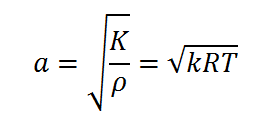# Speed of sound using Newton-Laplace Equation Calculator

This CalcTown calculator calculates the speed of sound using Newton-Laplace equation at a particular temperature.

Here, the speed of sound will be calculated by taking its ratio with speed of sound at T=300 K

K

#### Result

m/swhere

K is th Bulk Modulus of air

k is the adiabatic index of air (~1.4)

R is the Universal Gas constant